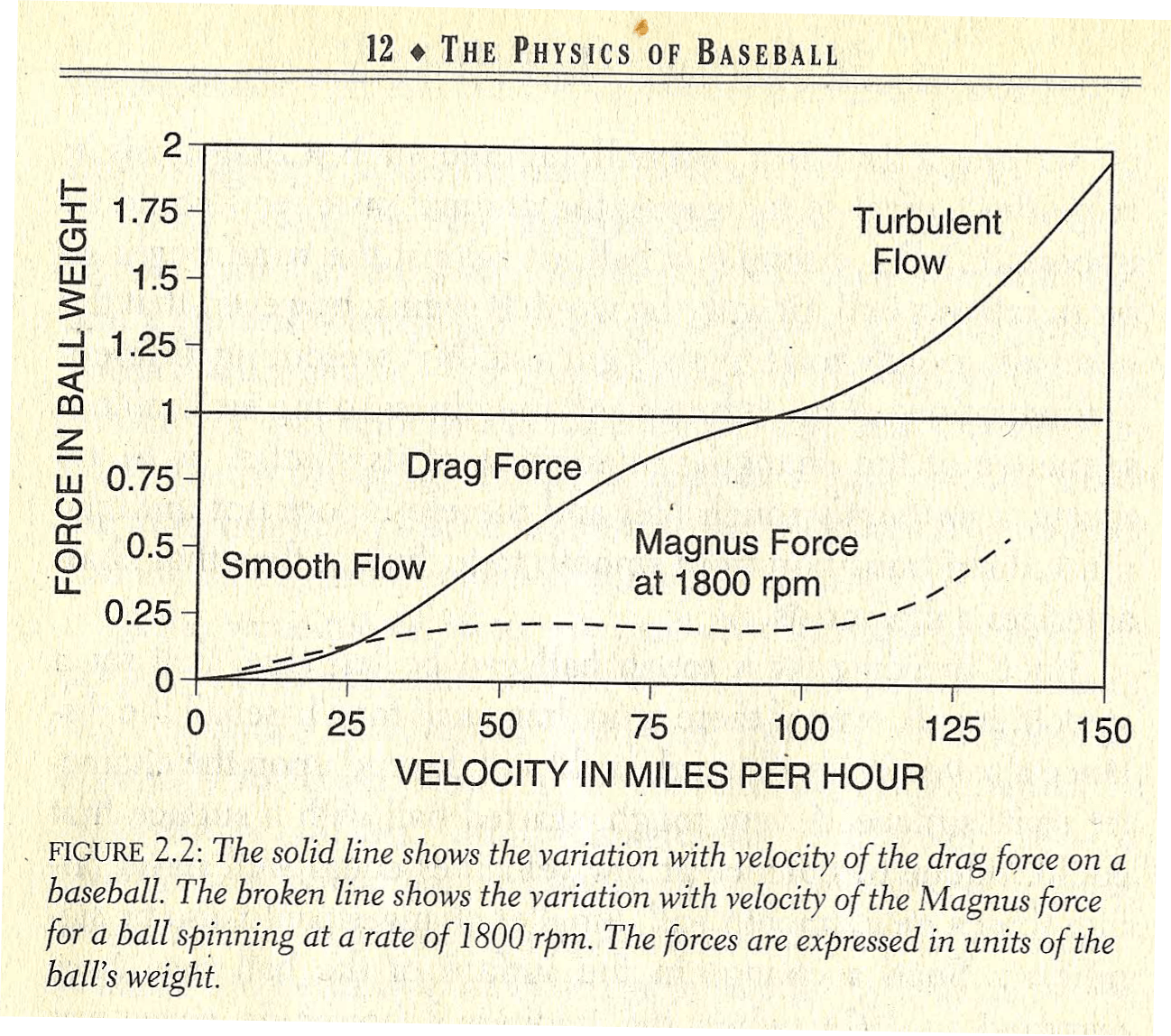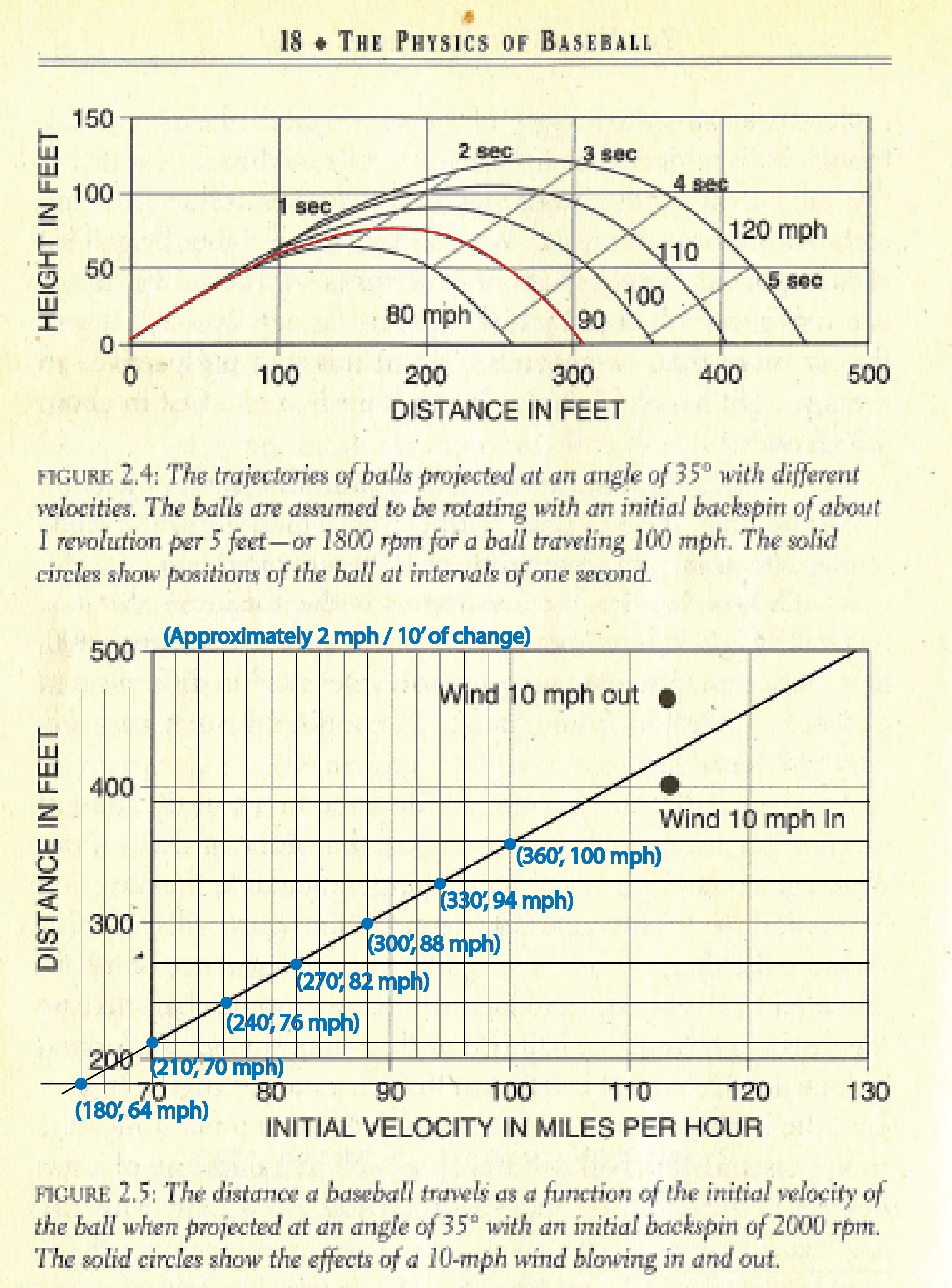# Theoretical

From Dr. Robert Adair’s The Physics of Baseball,

“Whether it is projected by arm or bat, the distance a ball will travel can be calculated using the simple ballistic relations governing a body in flight and taking the retarding drag force in the direction of motion and the Magnus force, normal to the direction of motion, from values shown in Figure 2.2.  Typical trajectories are shown in Figure 2.4, and a graph of the maximum distance vs. the initial ball velocity is shown in Figure 2.5.From Figure 2.4, the maximum distance is obtained with balls projected at an initial angle of about 35° from the horizontal, though balls projected at 30° or 40° travel almost as far.  Note that the ball falls at a rather steep angle at the end of its flight; the trajectories are not symmetric.

Obviously these trajectories and maximum distances depend upon the drag and the drag coefficients, which are imperfectly known.  Assuming an uncertainty of 10% in the drag coefficients at high velocities, the uncertainty in the maximum distance of a ball’s flight will be about 3%, or about 12 feet for a 400 foot home run.  Since we are primarily interested in differences in distance that follow from changes in conditions, such an accuracy is acceptable.

As a ball is thrown or batted for distance in a real situation, it usually has appreciable backspin (on the order of 2000 rpm), which generates a significant force perpendicular to the direction of motion, generally upward.  Though the spin will probably increase the drag on the ball slightly, as a consequence of the lift provided by backspin, the Magnus force will somewhat increase the distance traveled by balls hit or thrown at smaller angles and reduce the distance of balls hit or thrown at larger angles.  In general, the backspin can be expected to produce a modest increase in the distance the ball will travel, as well as decrease of a few degrees in the optimum angle of projection.  According to my calculations, a ball launched without backspin at an angle of 35° traveling 385 feet would travel roughly 400 feet if the backspin were about 2000 rpm.  There are two counteracting effects: the backspin causes the ball to stay in the air longer, thus increasing the distance it travels; but the spin also increases the drag, hence decreasing the flight distance.  Since neither effect is known precisely, the magnitude of the backspin effect is uncertain.

Since the backspin itself depends in detail on the exact manner in which the ball is thrown or struck by the bat, to calculate the representative trajectories and distances given in Figures 2.4 and 2.5, I have assumed a backspin of about 1 rotation per 5 feet of flight.  Thus the rotation rate is proportional to the velocity.  For a ball traveling at 100 mph, that rate will be about 1800 rpm.”

I have modified Dr. Adair’s Figure 2.5 below to show approximate maximum distances for initial velocities at common distances used in long toss programs.  It is important to understand what Dr. Adair said previously about Figure 2.5: “the maximum distance vs. the initial ball velocity”.  That means that initial velocities may be higher than that predicted if using distance as the measure of ball release velocity.  For example, if a long toss ball is thrown 300 feet, then the minimum initial velocity is approximately 88 mph assuming ideal trajectories and spin rates.  If the initial launch conditions are far from optimal such as much higher launch angle and/or much different conditions and the ball still travels 300 feet, then the initial velocity may be higher than 88 mph.  Or in other words, a ball thrown with an initial velocity of 88 mph with less than ideal launch conditions, then it will travel less than 300 feet.  Also, it must be noted again that these charts all assume no wind which will also affect the distance traveled for a given initial velocity.This can more easily be visualized by looking at Figure 2.4, which shows typical trajectories for balls launched with near ideal launch conditions of 35° and 1800 rpm backspin.  If a ball was launched with an angle of say 50 degrees and/or increased backspin and still travels 300 feet, then it may have an initial velocity closer to 90-92 mph.  While these are just estimates based on Dr. Adair’s calculations and charts, it is important to understand what these charts signify for purposes of estimating the exit velocity from the pitcher’s hand during long toss.